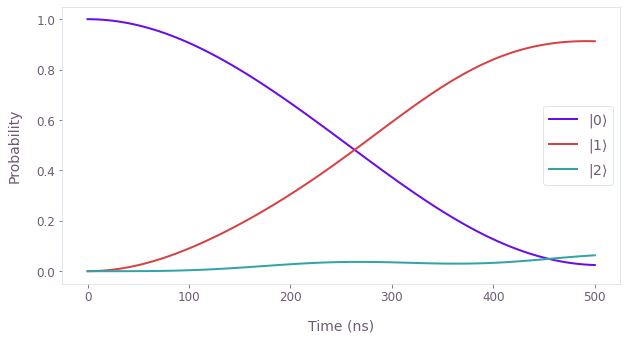•Boulder Opal
• User guides
• Use QuTiP operators in graphs

# How to use QuTiP operators in graphs

Incorporate QuTiP objects and programming syntax directly into graphs

It is possible to use Boulder Opal with QuTiP operators. This is possible in graph execution, where every operator can be replaced by QuTiP's Qobj. In case QuTiP is not available, these operators are NumPy arrays.

## Example: QuTiP operators in a graph-based simulation of a single qubit with leakage

In this example we to simulate the dynamics of a single qubit subject to leakage to an additional level. The resulting qutrit is treated as an oscillator (truncated to three levels) with an anharmonicity of $\chi$, described by the Hamiltonian:

\begin{align} H(t) = & \frac{\chi}{2} (a^\dagger)^2 a^2 + \frac{\Omega(t)}{2} a + \frac{\Omega^*(t)}{2} a^\dagger, \end{align}

where $a = \left|0 \right\rangle \left\langle 1 \right| + \sqrt{2} \left|1 \right\rangle \left\langle 2 \right|$ is the lowering operator and $\Omega(t)$ is a time-dependent Rabi rate.

In the example code below we illustrate how the noise-free infidelity $\mathcal{I}_0$ can be extracted from the coherent simulation results. To do this, we choose as target an X gate between the states $\left| 0 \right\rangle$ and $\left| 1 \right\rangle$. Notice that this target is not unitary in the total Hilbert space, but is still a valid target because it is a partial isometry—in other words, it is unitary in the subspace whose basis is $\{ \left| 0 \right\rangle, \left| 1 \right\rangle \}$.

Note the use of QuTiP to define operators where convenient in defining the relevant graph nodes.

import matplotlib.pyplot as plt
import numpy as np
from qctrlopencontrols import new_primitive_control
from qctrlvisualizer import get_qctrl_style, plot_population_dynamics

from qctrl import Qctrl

# Start a Boulder Opal session
qctrl = Qctrl()

plt.style.use(get_qctrl_style())
# Define system parameters
chi = 2 * np.pi * 3 * 1e6  # Hz
omega_max = 2 * np.pi * 1e6  # Hz
total_rotation = np.pi

# Define pulse using pulses from Q-CTRL Open Controls
pulse = new_primitive_control(
rabi_rotation=total_rotation,
azimuthal_angle=0.0,
maximum_rabi_rate=omega_max,
name="primitive",
)

sample_times = np.linspace(0, pulse.duration, 100)

try:
import qutip as qt

# Define matrices for the Hamiltonian operators
a = qt.destroy(3)
n = qt.num(3)
ad2a2 = n * n - n

# Define the target
target_operation = qt.Qobj(np.array([[0, 1, 0], [1, 0, 0], [0, 0, 0]]))

# Define the initial state
initial_state = qt.basis(3)

graph = qctrl.create_graph()

except ModuleNotFoundError:
graph = qctrl.create_graph()

# Define matrices for the Hamiltonian operators
a = graph.annihilation_operator(3)
n = graph.number_operator(3)
ad2a2 = n @ n - n

# Define the target
target_operation = np.array([[0, 1, 0], [1, 0, 0], [0, 0, 0]])

# Define the initial state
initial_state = graph.fock_state(3, 0)[:, None]

pass

# Define the anharmonic term
anharmonic_drift = 0.5 * chi * ad2a2

# Define Rabi drive term
rabi_rate = graph.pwc(
durations=pulse.durations,
values=pulse.rabi_rates * np.exp(1j * pulse.azimuthal_angles),
)
rabi_drive = graph.hermitian_part(rabi_rate * a)

hamiltonian = anharmonic_drift + rabi_drive

# Calculate the time evolution operators
unitaries = graph.time_evolution_operators_pwc(
hamiltonian=hamiltonian, sample_times=sample_times, name="time_evolution_operators"
)

# Calculate the infidelity
infidelity = graph.unitary_infidelity(
unitary_operator=unitaries[-1], target=target_operation, name="infidelity"
)

evolved_states = unitaries @ initial_state
evolved_states.name = "evolved_states"

# Run simulation
graph_result = qctrl.functions.calculate_graph(
graph=graph,
output_node_names=["evolved_states", "infidelity", "time_evolution_operators"],
)

# Extract and print final infidelity
print(f"Noise-free infidelity at end: {graph_result.output['infidelity']['value']:.1e}")

# Extract and print final time evolution operator
print("Time evolution operator at end:")
print(np.round(graph_result.output["time_evolution_operators"]["value"][-1], 3))

# Extract and plot state populations
state_vectors = graph_result.output["evolved_states"]["value"]
plot_population_dynamics(
sample_times,
{rf"$|{state}\rangle$": np.abs(state_vectors[:, state]) ** 2 for state in range(3)},
)
Your task calculate_graph (action_id="1618606") has completed.
Noise-free infidelity at end: 8.7e-02
Time evolution operator at end:
[[ 0.057-0.145j  0.235-0.926j -0.088+0.235j]
[ 0.235-0.926j -0.067+0.187j -0.193+0.102j]
[-0.088+0.235j -0.193+0.102j -0.825+0.456j]]This notebook was run using the following package versions. It should also be compatible with newer versions of the Q-CTRL Python package.

PackageVersion
Python3.10.8
matplotlib3.6.3
numpy1.23.1
qutip4.7.1
scipy1.10.0
qctrl20.2.0
qctrl-commons17.10.0
qctrl-open-controls9.1.7
boulder-opal-toolkits2.0.0-beta.4
qctrl-visualizer4.6.0